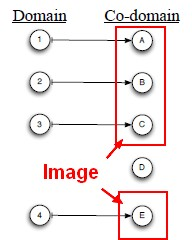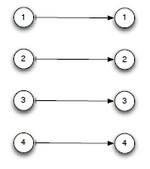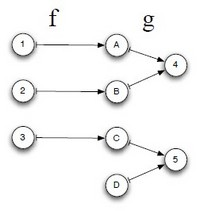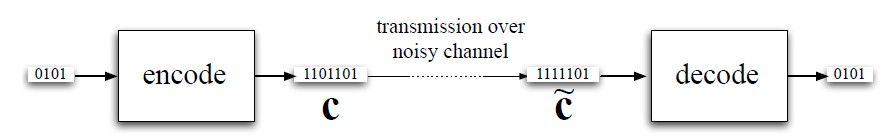# Linear Algebra - Function (Set)

## Definition

For each input element in a set $A$ , a function assigns a single output element from another set $B$ .

• $A$ is called the domain of the function
• $B$ is called the co-domain

!!!!!! Same as Set Theory - Function (Mathematical) !!!!!!

## Syntax

$f(A) = B$

or in Mathese:

$f : A \rightarrow B$

## Example

• The function that doubles its input:
f({1, 2, 3,...}) = {(1,2),(2,4),(3,6),(4,8),....}


• The function that multiplies the numbers forming its input:
f({1, 2, 3,...}x{1, 2, 3,...}) = {((1,1),2),((1,2),4),...,((2,2),4),(2,3),6), ....}


• Caesar’s Cryptosystem

Each letter is mapped to one three places ahead, wrapping around, so MATRIX would map to PDWULA. The function mapping letter to letter can be written as:

{('A','D'),('B','E'),('C','F'),('D','G'),...,('W','Z'),('X','A'),('Y','B'),('Z','C')}



Both the domain and the co-domain are {A,B, …,Z}

## Image

for a function $f : V \mapsto W$ , the image of f is the set of all images of elements of the domain: $${f (v) : v \in V}$$

The image of a function is the set of all images of inputs. Mathese:

lm f


The output of a given input is called the image of that input. The image of A under the function f is denoted f(A).

There might be elements of the co-domain that are not images of any elements of the domain.

Example
A function f : {1, 2, 3, 4} −> {'A','B','C','D','E'}
The image of f is lm f = {'A','B','C','E'}
'D' is in the co-domain but not in the image## Set

Set of functions: For sets F and D, $F^D$ denotes all functions from D to F.

For finite sets, the cardinality $|F^D| = |F|^{|D|}$

## Function

### Inverse

Functions f and g are functional inverses ifandare defined and are identity functions.

### Invertible

A function that has an inverse is invertible.

Invertible functions are:

Function Invertibility Theorem: A function f is invertible if and only if it is one-to-one and onto.

Linear-Function Invertibility Theorem: A function $f: V \mapsto W$ is invertible iff

• $\text{dim Ker f = 0 and dim lm f = dim W }$
• $\text{dim Ker f = 0 and dim V = dim W }$ (For f to be invertible, need dim V = dim W)

Extracting an invertible function:

Let $A = \begin{bmatrix} \begin{array}{r|r|r} 1 & 2 & 1 \\ 2 & 1 & 1 \\ 1 & 2 & 1 \\ \end{array} \end{bmatrix}$ and define $f : \mathbb{R}^3 \mapsto \mathbb{R}^3$ by $f (x) = Ax$

Define $W^* = lm f = Col A = Span \{[1, 2, 1], [2, 1, 2], [1, 1, 1]\}$

One basis for $W^*$ is:

• $w_1 = [0, 1, 0]$ ,
• $w_2 = [1, 0, 1]$

Pre-images for $w_1$ and $w_2$ :

• $v_1 = [\frac{1}{2},−\frac{1}{2},\frac{1}{2}]$
• and $v_2 = [−\frac{1}{2},\frac{1}{2},\frac{1}{2}]$

for then $A.v_1 = w_1$ and $A.v_2 = w_2$

Let $V^* = Span \{v_1, v_2\}$ Then $f : V^* \mapsto lm f$ is onto and one-to-one and therefore invertible.

### Relationship

#### One-to-one

$f : V \rightarrow W$ is one-to-one if f (x) = f (y) implies x = y

One-to-One Lemma: A linear function is one-to-one if and only if its kernel is a trivial vector space. Equivalent: if its kernel has dimension zero.

f is one-to-one iff $\href{dimension}{dim} \text{ } \href{#kernel}{Ker} \text{ } f = 0$

#### Onto

$f : V \rightarrow W$ is onto if for every $z \in F$ there exists an a such that $f(a) = z$

f is onto if its image equals its co-domain

For any linear function $f : V \mapsto W$ , f is onto if $\href{dimension}{dim} \text{ } \href{#image}{lm} f = \href{dimension}{dim} \text{ } W$

Discover More(Function | Operator | Map | Mapping | Transformation | Method | Rule | Task | Subroutine)

Section computable function. A function is a callable unit that may be called: a procedure, a subrontine a routine, a method (belong to an objectmacrocomputablalgorithreusable blocargumentdevelopment...(Function|Operator) - Associative Property

An operator or functionop is associative if the following holds: The importance of this to parallel evaluation can be seen if we expand this to four terms: So we can evaluate (a op b) in parallel...Analytic (Data Analyst)

A Data Analyst sees what data he has. A Data Scientist imagines what data he is lacking. Information analysis provides insight into : the past the present and the future of the business. See...Function - Identity

An identity function is an algebraic law that defines when the output of the function is equal to the input. identity function. For any domain D: each domain element d map to itself: Addition...Linear Algebra - Basis of a Vector Space

A basis for vector space V is a linearly independent set of generators for V. Thus a set S of vectors of V is a basis for V if S satisfies two properties: Property B1 (Spanning) Span S = V, and Property...Linear Algebra - Dimension of a vector space

The dimension of a vector space V is the size of a basis for that vector space written: dim V. rank If U is a subspace of W then D1: (or ) and D2: if then Example: Suppose V = Span...Linear Algebra - Function composition

Functions + and the functional composition of f and g is the function: defined by: Example is the Caesar cypher Associativity of function composition:...Linear Algebra - Linear Function (Weighted sum)

f is a linear function if she is defined by where: M is an R x C matrix and A Linear function can be expressed as a matrix-vector product: If a function can be expressed as a matrix-vector product...Linear Algebra - Linear binary code

In a linear binary code, the set C of codewords is a vector space over GF(2). In such a code, there is a matrix H, called the check matrix, such that C is the null space of H. When the Receiver receives...Linear Algebra - Matrix

The Traditional notion of a matrix is: a two-dimensional array a rectangular table of known or unknown numbers One simple role for a matrix: packing together a bunch of columns or rows Matrix...